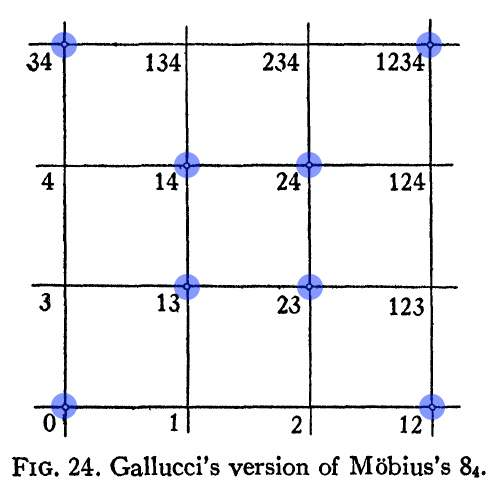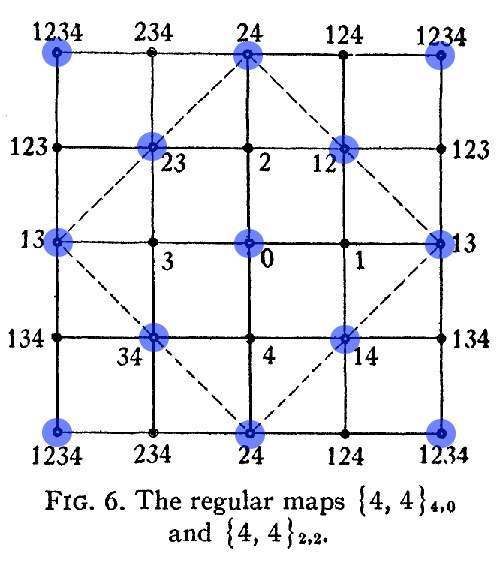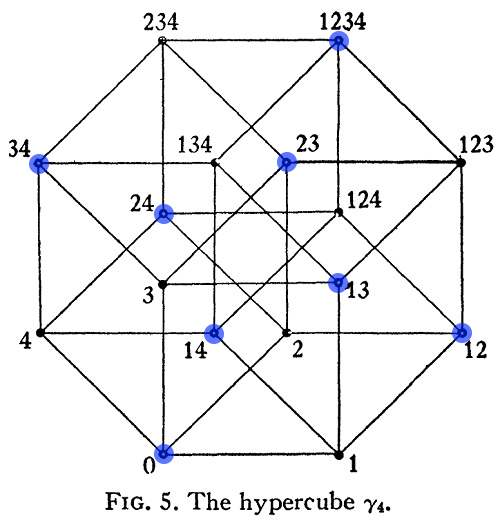# Log24

## Thursday, March 26, 2015

### The Möbius Hypercube

Filed under: General,Geometry — Tags: , — m759 @ 12:31 AM

The incidences of points and planes in the
Möbius 8 configuration (8 points and 8 planes,
with 4 points on each plane and 4 planes on each point),
were described by Coxeter in a 1950 paper.*
A table from Monday's post summarizes Coxeter's
remarks, which described the incidences in
spatial terms, with the points and planes as the vertices
and face-planes of two mutually inscribed tetrahedra —Monday's post, "Gallucci's Möbius Configuration,"
may not be completely intelligible unless one notices
that Coxeter has drawn some of the intersections in his
Fig. 24, a schematic representation of the point-plane
incidences, as dotless, and some as hollow dots.  The figure,
"Gallucci's version of Möbius's 84," is shown below.
The hollow dots, representing the 8 points  (as opposed
to the 8 planes ) of the configuration, are highlighted in blue.Here a plane  (represented by a dotless intersection) contains
the four points  that are represented in the square array as lying
in the same row or same column as the plane.

The above Möbius incidences appear also much earlier in
Coxeter's paper, in figures 6 and 5, where they are shown
as describing the structure of a hypercube.

In figures 6 and 5, the dotless intersections representing
planes have been replaced by solid dots. The hollow dots
have again been highlighted in blue.Figures 6 and 5 demonstrate the fact that adjacency in the set of
16 vertices of a hypercube is isomorphic to adjacency in the set
of 16 subsquares of a square 4×4 array, provided that opposite
sides of the array are identified, as in Fig. 6. The digits in
Coxeter's labels above may be viewed as naming the positions
of the 1's in (0,1) vectors (x4, x3, x2, x1) over the two-element
Galois field.  In that context, the 4×4 array may be called, instead
of a Möbius hypercube , a Galois tesseract .

*  "Self-Dual Configurations and Regular Graphs,"
Bulletin of the American Mathematical Society,
Vol. 56 (1950), pp. 413-455

The subscripts' usual 1-2-3-4 order is reversed as a reminder
that such a vector may be viewed as labeling a binary number
from 0  through 15, or alternately as labeling a polynomial in
the 16-element Galois field GF(24).  See the Log24 post
Vector Addition in a Finite Field (Jan. 5, 2013).

## No Comments

No comments yet.

RSS feed for comments on this post.

Sorry, the comment form is closed at this time.

Powered by WordPress